# Time index details

## Reference to earlier time

Time - k in the expression var[Time - k] refers to the position of the elements in the Time index, not values of Time.

For example, if Time equals [1990, 1994, 1998, 2002, 2006], then the value of Gasprice[Time - 3] in year 2006 would refer to the price of gasoline in 1994, not 2003. When you refer to the Time variable directly, not as an index, the expression refers to the values of Time. For example, the expression (Time - 3) in 2006 is 2003.

The offset, «k», can be an expression, and might even be indexed by Time. When «k» is indexed by Time, then the offset varies at different points in Time. However, Slice(k, Time, t) must be between 1 and t - 1. It must be positive since the expression is not allowed to depend on values in the future (that have not yet been computed). It must be less than t - 1 since the expression cannot depend on values “before the beginning of time.”

## Defining time

There are common three ways to define the Timeindex, each of which has different advantages:

• Sequence (the preferred method)
• List (numeric)
• List of labels (text)

### Time as a sequence

Using the Sequence function is the easiest way to define Timewith equal intervals (see Expression view and Defining a variable as an edit table). The numeric values for Timecan be used in other expressions.

Example: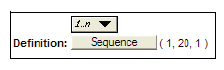It is also common to use a date sequence. A couple common examples are

• Step size of one day: Sequence( 1-Jan-2017, 31-Dec-2020 )
• Weekdays only: Sequence(1-Jan-2017, 31-Dec-2020, dateUnit:'wd')
• Step size of one month: Sequence(1-Jan-2017, 31-Dec-2020, dateUnit:'M')
• Set Number Format / Date for Time to Custom = yyyy-MM
• Each element is a date-number equal to the 1st of the month. Date arithmetic and date functions can be directly applied to the elements.

### Time as a list (numeric)

When Timeis defined as a numeric list, it usually consists of increasing numbers. The intervals between entries can be unequal, and the values for Timecan be used in other expressions.

Example: Time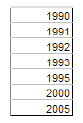When you use time periods that differ by a value other than 1, typing (Time-1) won’t provide the value of the previous time period. You can use the syntax x[Time-1] if you want to utilize a variable indexed by Time, but if you want to perform an operation that depends on the difference in time between the current time period and the last one, you must first create a node that uncumulates the Timeindex.

YearsPassed: Uncumulate(Time,Time)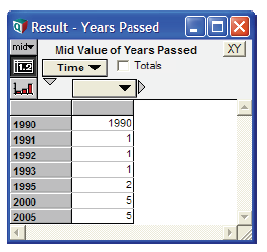Now you can include this node in a dynamic expression that depends on the time between time periods. The following definition is equivalent to the Dynamic() definition but allows for changes in time period increments.

Gasprice := Dynamic(1.2, Gasprice[Time - 1]*1.05^YearsPassed) →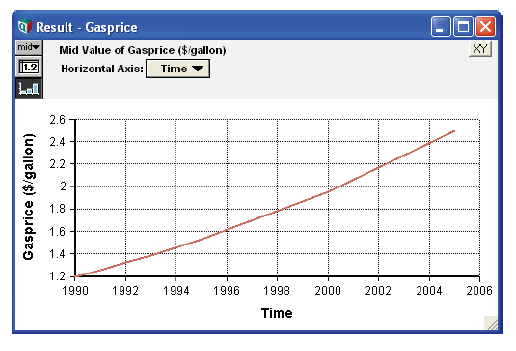### Time as a list of labels (text)

When Timeis defined as a list of labels, Timevalues cannot be used in other expressions as numbers.

The resulting graph of any Dynamic() function, with the x-axis set to Time, shows the labels at equal x-axis intervals.

Example: Time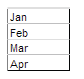Gasprice := Dynamic(1.2, Gasprice[Time - 1]*1.05) →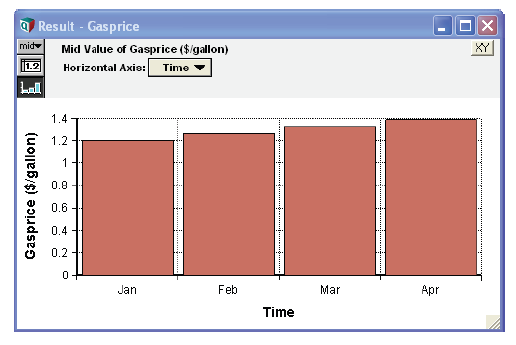## Using Time in a model

You can use Timelike any index variable; you can change only its title and definition. To include the Timenode on a diagram:

1. Open the Object window for Timeby selecting Edit Time from the Definition menu.
2. Select Make Alias from the Object menu (see An alias is like its original).

When the Time node displays on a diagram, arrows from Timeto all dynamic variables display by default.